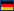The corresponding C++ feature to Delphi's anonymous methods are lambda expressions. The translation is quite straight forward:

An anonymous method type can be declared as a reference to a method. It becomes in C++ to a std::function type:

```type
TFuncOfInt = reference to function(x: Integer): Integer;
```

```var
begin
adder :=  function(X: Integer) : Integer
begin
Result := X + Y;
end;
```
##### ->

```typedef std::function TFuncOfInt;
```

``` TFuncOfInt adder;
adder = [&](int X) -> int {
int result = 0;
result = X + Y;
return result;
};
```

As well as anonymous methods can be assigned to a method reference (see above), a normal method can be assigned to it. In C++ this is done by means of std::bind. The expression of this assignment looks quite complicated however, because std::placeholders are needed to represent unbound variables.

```type
TMethRef = Reference to procedure(X: Integer);
```

```TAn3Class = class(TObject)
procedure method(X: Integer);
end;
```

```procedure Test;
var
m: TMethRef;
i: TAn3Class;
begin
// ...
m := i.method;
end;
```

##### ->

```typedef std::function TMethRef;
```

```class TAn3Class : public System::TObject
{
typedef System::TObject inherited;
public:
void method(int X);
public:
TAn3Class() {}
};
```

```void Test()
{
TMethRef m;
TAn3Class* i = nullptr;
// ...
m = std::bind(&TAn3Class::method, i, std::placeholders::_1);
}
```Deutsch

 Latest News 11/15/19 DelphiXE2Cpp11 nifty class references offer [more...] 10/16/19 Delphi2C# beta offer [more...]